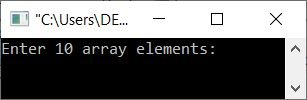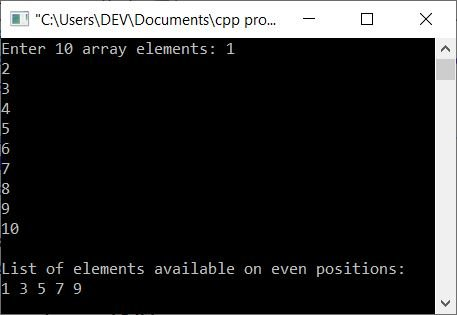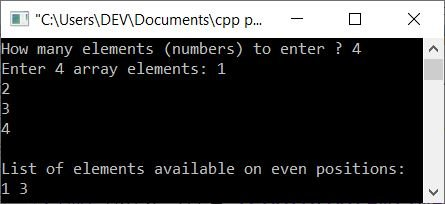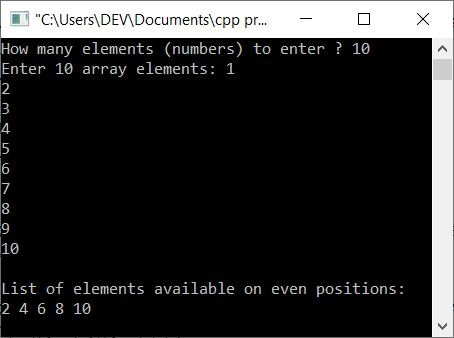# C++ Program to Print All Elements Available in Even Positions

This article provides some programs in C++ that find and print elements or numbers available on even positions in an array. Here is a list of programs provided here:

## Print elements in even index positions

The question is: write a C++ program that receives 10 elements for the array to find and print all elements available in even positions. Here is its answer:

```#include<iostream>

using namespace std;
int main()
{
int arr, i;
cout<<"Enter 10 array elements: ";
for(i=0; i<10; i++)
cin>>arr[i];
cout<<"\nList of elements available on even positions:\n";
for(i=0; i<10; i++)
{
if(i%2==0)
cout<<arr[i]<<" ";
}
cout<<endl;
return 0;
}```

The snapshot given below shows the initial output produced by the above C++ program on printing all even-positioned elements:Now supply the input, say 1, 2, 3, 4, 5, 6, 7, 8, 9, 10, as ten numbers, and press the ENTER key to find and print all elements or numbers available on even indices, like shown in the snapshot given below:The above program works only on 10 elements. That is, to allow the user to work with the required number of elements, here is another program created after modifying the above one:

```#include<iostream>

using namespace std;
int main()
{
int arr, i, n;
cout<<"How many elements (numbers) to enter ? ";
cin>>n;
cout<<"Enter "<<n<<" array elements: ";
for(i=0; i<n; i++)
cin>>arr[i];
cout<<"\nList of elements available on even positions:\n";
for(i=0; i<n; i++)
{
if(i%2==0)
cout<<arr[i]<<" ";
}
cout<<endl;
return 0;
}```

Here is its sample run with user input 4 as size and 1, 2, 3, 4 as its elements:Note: Since the maximum size defined for the array arr in the above program is 100, As a result, do not provide a size greater than this number. But I've created another program that defines the size of the array arr dynamically, as shown in the upcoming program.

### Print elements on even indexes using the second array

This program uses another array to store all the elements available on even index numbers, one by one.

```#include<iostream>

using namespace std;
int main()
{
int n, i, j=0;
cout<<"How many elements (numbers) to enter ? ";
cin>>n;
int arr[n], eve[n];
cout<<"Enter "<<n<<" array elements: ";
for(i=0; i<n; i++)
{
cin>>arr[i];
if(i%2==0)
{
eve[j] = arr[i];
j++;
}
}
cout<<"\nList of elements available on even positions:\n";
for(i=0; i<j; i++)
cout<<eve[i]<<" ";
cout<<endl;
return 0;
}```

This program produces exactly the same output as the previous program.

## Print Element in Even Position, Standard Position

This is the final program in this article, and it finds and prints all of the elements that are available in even position.

```#include<iostream>

using namespace std;
int main()
{
int n, i, j=0;
cout<<"How many elements (numbers) to enter ? ";
cin>>n;
int arr[n], eve[n];
cout<<"Enter "<<n<<" array elements: ";
for(i=0; i<n; i++)
{
cin>>arr[i];
if((i+1)%2==0)
{
eve[j] = arr[i];
j++;
}
}
cout<<"\nList of elements available on even positions:\n";
for(i=0; i<j; i++)
cout<<eve[i]<<" ";
cout<<endl;
return 0;
}```

Here is its sample run with user input of 10 as size, and again 1, 2, 3, 4, 5, 6, 7, 8, 9, 10 as ten array elements:Note: The only difference between this program and the previous one is that the following code has been replaced:

`if(i%2==0)`

with the code given below:

`if((i+1)%2==0)`

C++ Quiz

« Previous Program Next Program »